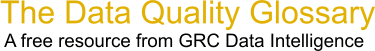Choose Index below for a list of all words and phrases defined in this glossary.

# Bayes' Theorum

index | Index

## Bayes' Theorum - definitions

Bayes' Theorum - A formula to calculate conditional probabilities by relating the conditional and marginal probability distributions of random variables.

[Category=Quality ]

Source: American Society for Quality, 07 September 2010 08:24:22, http://www.asq.org/glossary/These advertisers support this free service

Bayes' theorem - [statistics] A theorem developed by English mathematician Thomas Bayes (1702-1761) about conditional probability. It states that the probability of a given event, given the original data and some new data, is proportional to the probability of the event given the original data only, and the probability of the new data given the original data and the event.

[Category=Geospatial ]

Source: esri, 29 January 2012 15:03:14, http://support.esri.com/en/knowledgebase/GISDictionary/term/abbreviationData Quality Glossary.  A free resource from GRC Data Intelligence. For comments, questions or feedback: dqglossary@grcdi.nl